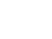# Exporting Acoustic Data to Matlab

Andy Todd

Posted on 01.11.2023 22:19

Hi all - hope you are well.

We are trying to export some acoustic measurement data from Dewesoft into Matlab for further analysis.

We have had slight success using Dewesoft wav export and importing this to Matlab - however the measured values are then incorrect and are arbitrary, so it is not possible to accurately calculate fft etc.

We have also tryed exporting in Matlab format, but this also does not seem correct - see image attached.

1. Dewesoft data: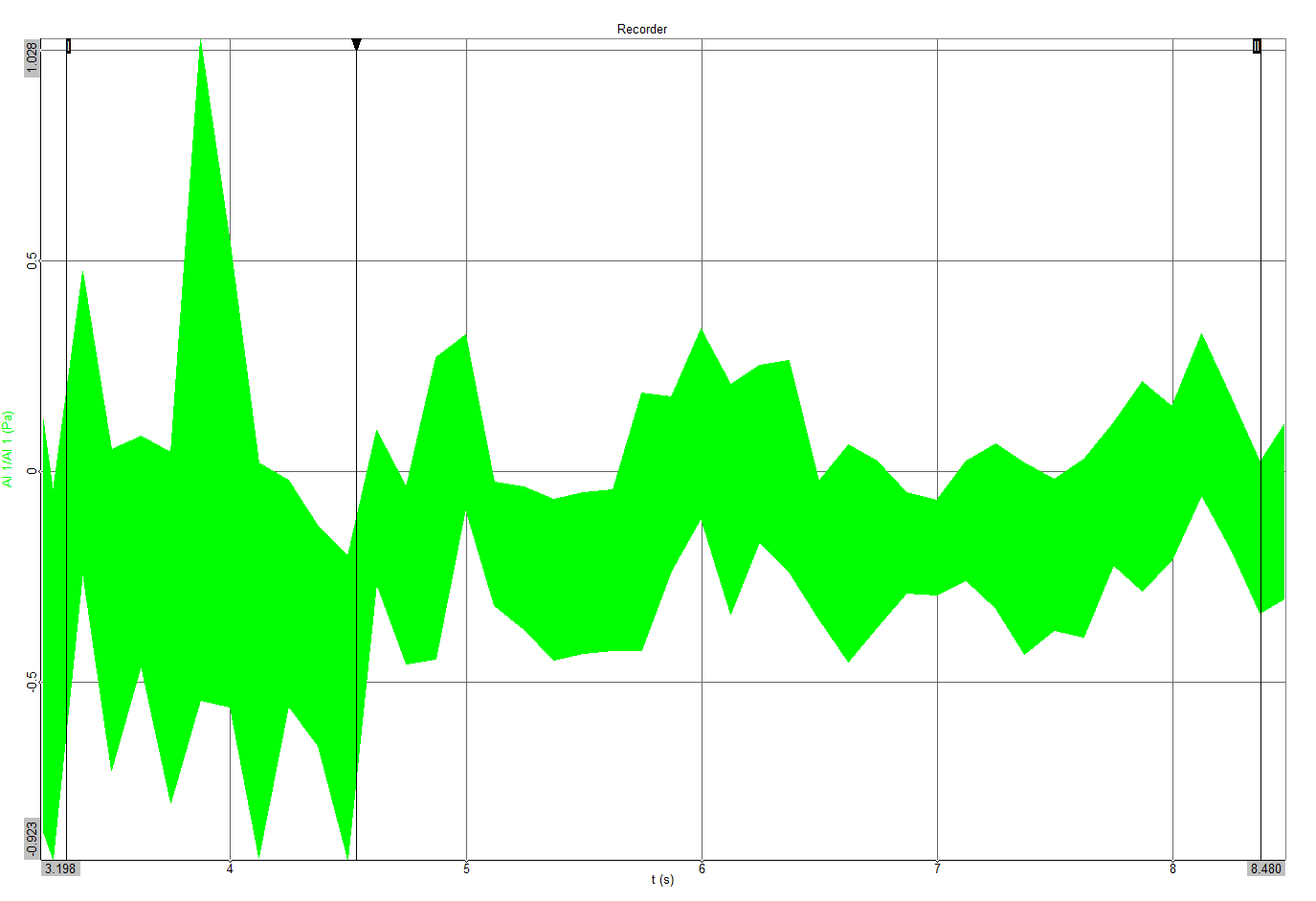2. As shown in Matlab following export from dewesoft in matlab format.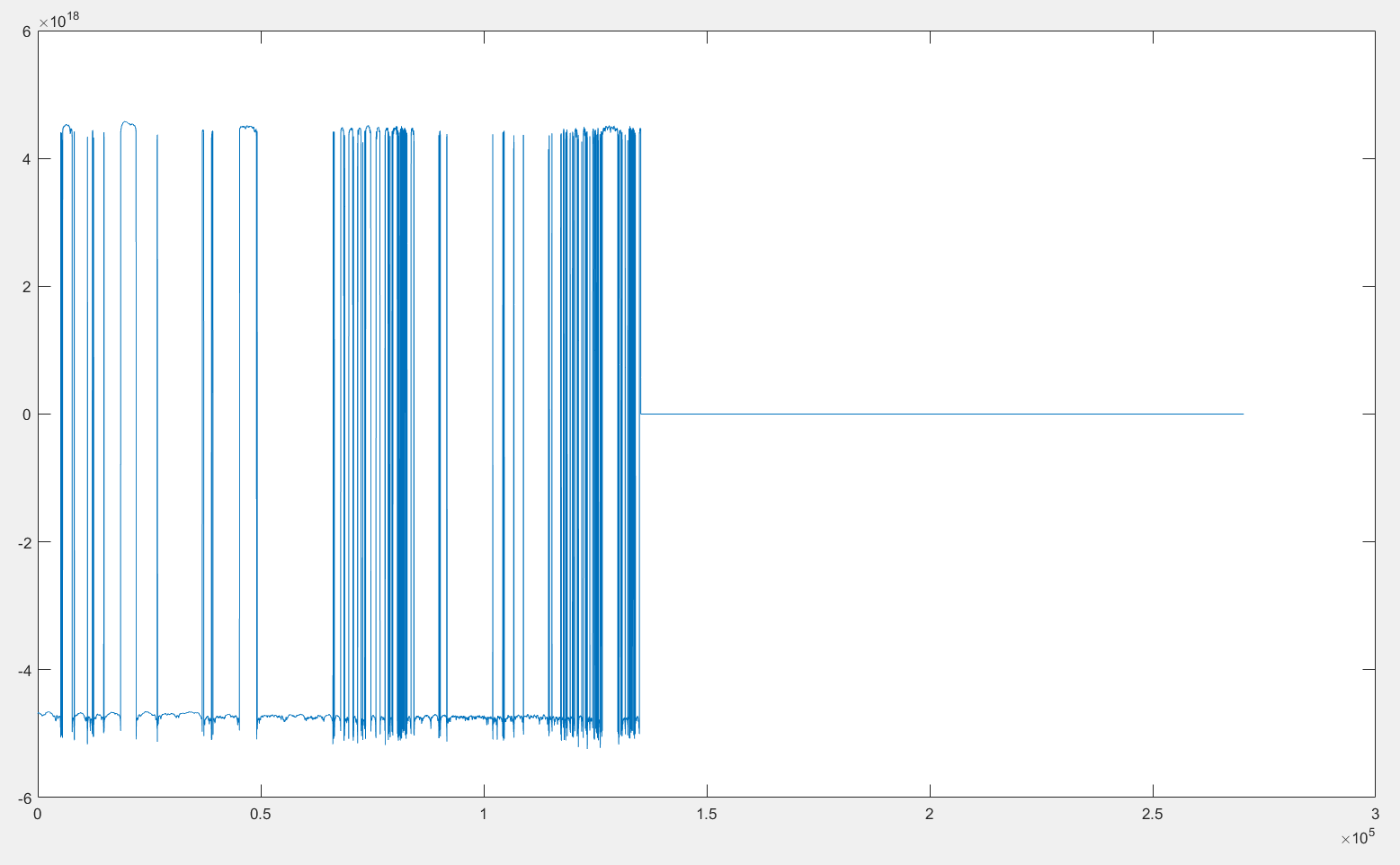So basically we are looking for a method to get the raw data into Matlab so that FFT, CPB etc can be calculated with correct values.

Hope that makes sense!

Andy

Pika Kreča Šmid

Posted on 02.11.2023 14:21

Dear Andy,

thank you for reaching out to us.

I have done a couple tests with my own datafile and DewesoftX version 2023.5. I can confirm that the following Matlab export is not correct. I believe it is a scaling issue, that appears to have been fixed with the 2023.6 RC version of DewesoftX.

As previously stated, I took my own file and exported the data to MATLAB, first in 2023.5, then in 2023.6. The .5 version produces a result, similar to yours. The .6 version, however produces correct values. You could, therefore try downloading the RC for the newest DewesoftX version.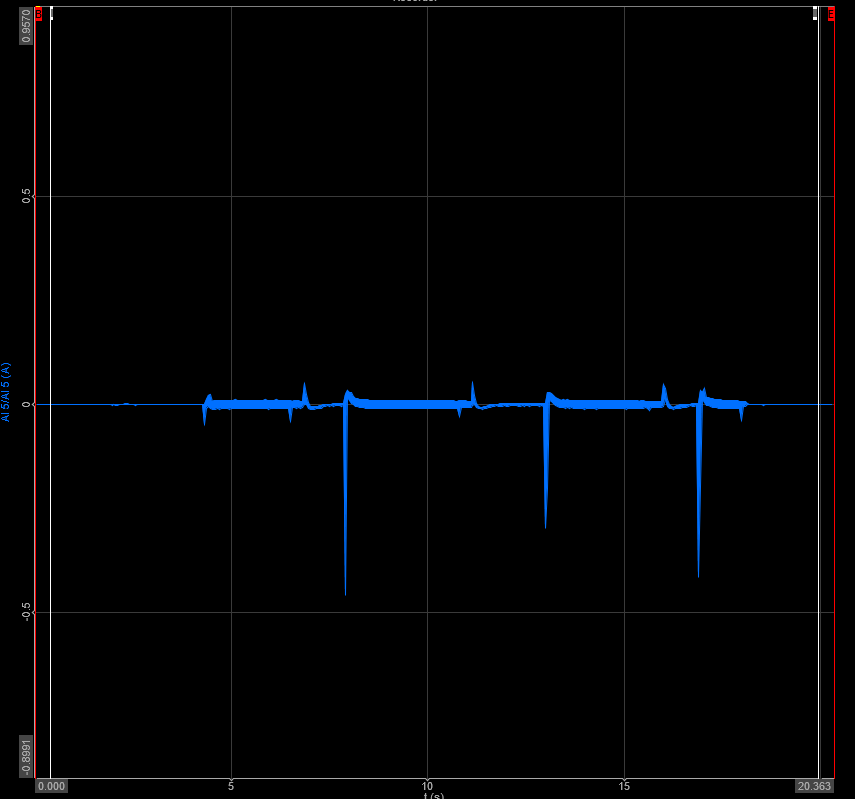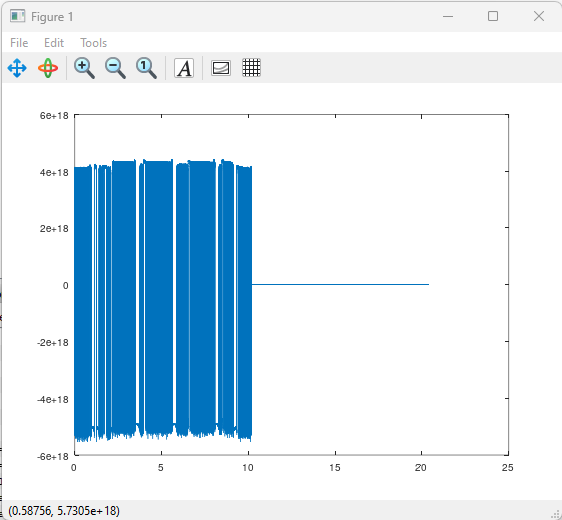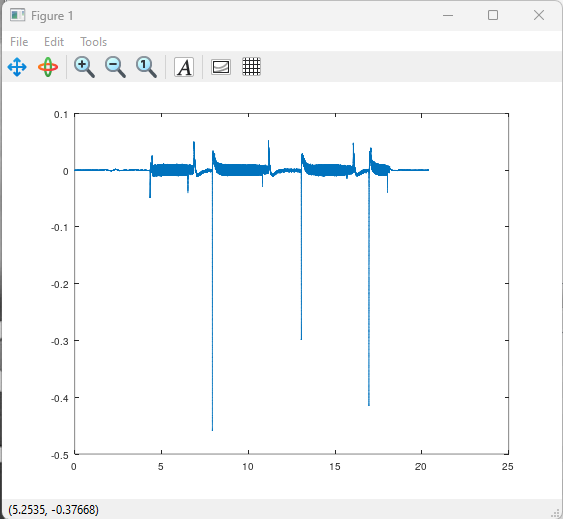I believe you could also work with .txt files, and .csv files.

''Spreadsheets, such as Microsoft® Excel® files, often contain a mix of numeric and text data as well as variable and row names. While the most common way to represent spreadsheet data in MATLAB® is by using a table, you can also represent spreadsheet data in MATLAB as timetables, matrices, or cell arrays.

Import data from spreadsheets either programmatically or interactively. Import programmatically using tailored importing functions, and further control how your data is imported using import options. Import interactively by using the Import Tool.''

On the image below is how I have exported my data from a .csv file, but I believe you could do the same for a plethora of other datafiles, such as .txt, or .xlsx.

My datafile was called Test_Tok1.csv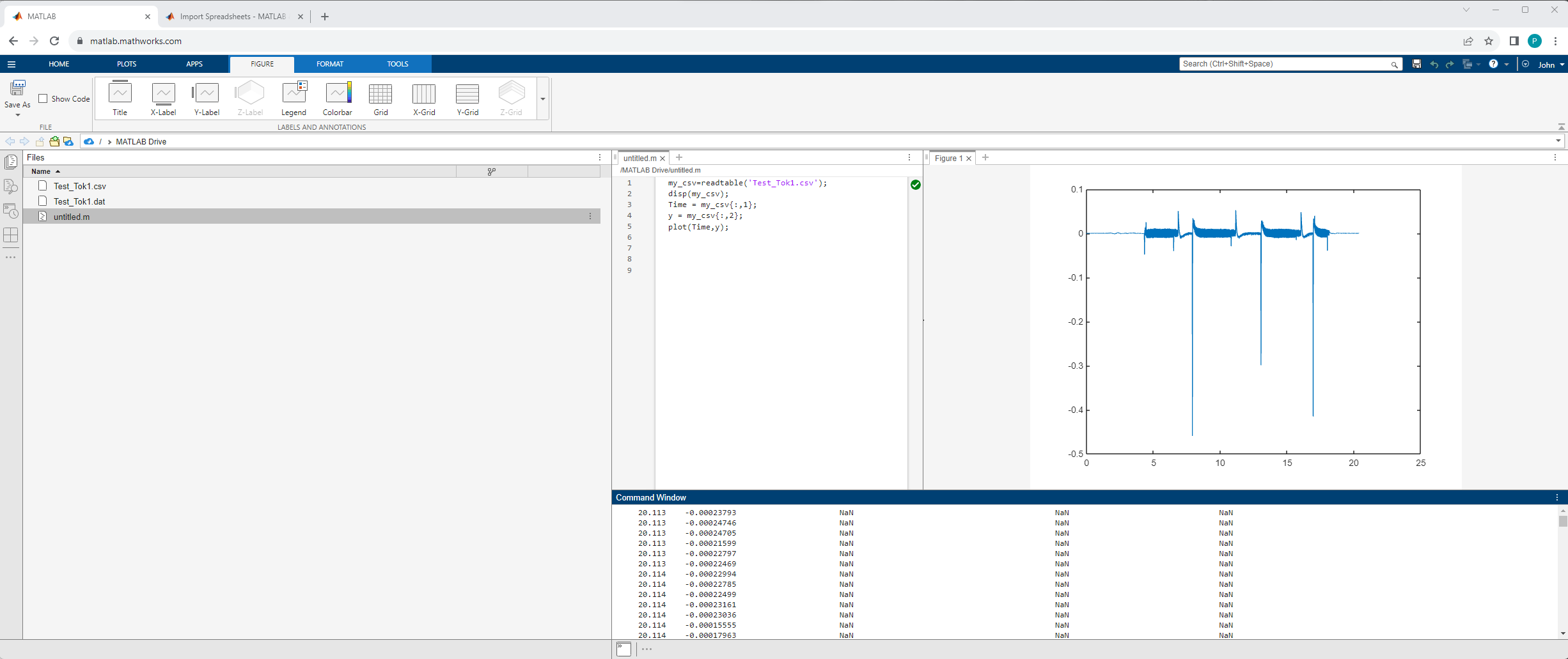We use the readtable(data) command to read the data. We then use the disp() command to display the data, just to confirm that everything works as it should. We set the first (time) column as Time, and the second (values) as y. In the end we plot a graph with a plot() command.

This should now give you correct values.

Here is the code I have used:

disp(my_csv);

Time = my_csv{:,1};

y = my_csv{:,2};

plot(Time,y);

I will also include my .csv file and my .dxd file, just in case you'd like to test this on my data.

Best regards,

Pika

Attached files:
Andy Todd

Posted on 02.11.2023 16:17

Hi Pika, thanks for getting back to me :)

You are right, the RC version does now export correctly to Matlab: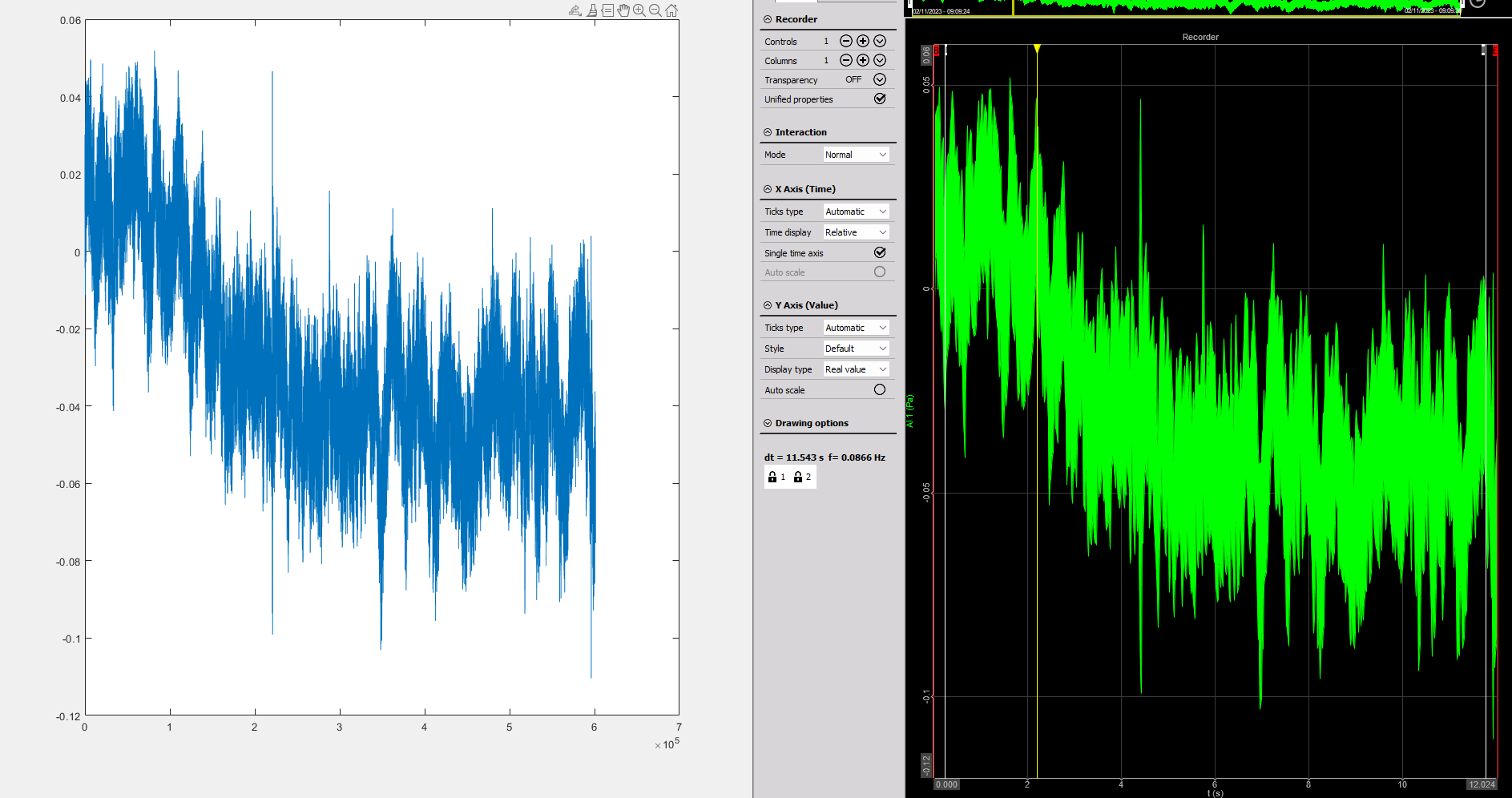The issue I have now is that I think there is a step missing - as when I try to convert this to an octave spectrum, Dewesoft obviously knows the scaling etc - whereas Matlab gives some different values: